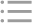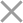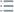## Fill out the form in 2 simple steps below:

Step 1

Step 2
** By clicking this button you agree to our Privacy Policy statementRequest our prices
--Select currency--
USD
EUR
* By clicking this button you agree to our Privacy Policy statementFree PVS‑Studio license for Microsoft MVP specialists
* By clicking this button you agree to our Privacy Policy statementTo get the licence for your open-source project, please fill out this form
* By clicking this button you agree to our Privacy Policy statementI am interested to try it on the platforms:
 Windows Linux macOS PVS-Studio for .NET Core JetBrains Rider
* By clicking this button you agree to our Privacy Policy statementMessage submitted.

Your message has been sent. We will email you at

check your Spam/Junk folder and click the "Not Spam" button for our message.
This way, you won't miss messages from our team in the future.

>
>
>
V3171. Potentially negative value is us…Introduction
Analyzing projects
On Windows
On Linux and macOS
Cross-platform
IDE
Build systems
Game Engines
Continuous use of the analyzer in software development
Deploying the analyzer in cloud Continuous Integration services
Managing analysis results
Analyzer diagnostics
General Analysis (C++)
General Analysis (C#)
General Analysis (Java)
Micro-Optimizations (C++)
Micro-Optimizations (C#)
Diagnosis of 64-bit errors (Viva64, C++)
Customer specific requests (C++)
MISRA errors
AUTOSAR errors
OWASP errors (C++)
OWASP errors (C#)
OWASP errors (Java)
Problems related to code analyzerContents

# V3171. Potentially negative value is used as the size of an array.

Apr 21 2021

The analyzer detected that a potentially negative value of a variable or expression might be used as the size of an array that is created.

Consider an example:

``````void ProcessBytes(byte[] bytes)
{
int length = BitConverter.ToUInt16(bytes, 0);
int[] newArr = new int[length - 2];
....
}``````

The value returned by the 'ToUInt16' method and assigned to the 'length' variable may be zero. This will happen if the first two bytes in the 'bytes' array are zero. This way, when you create the 'newArr' array, its length will be a negative value. This will result in the 'OverflowException' type exception.

Fixed version of the 'ProcessBytes' method with an additional check might look like this:

``````void ProcessBytes(byte[] bytes)
{
int length = BitConverter.ToUInt16(bytes, 0);

if (length < 2)
return;

int[] newArr = new int[length - 2];
....
}``````

Here is another code example. When you call the 'SubArray' method with a certain values of input arguments, a negative value can be used as a length of the array:

``````public byte[] GetSubArray(byte[] bytes)
{
return bytes.SubArray(4, 2);
}

public static T[] SubArray<T>(this T[] arr, int index, int length)
{
if (length < 0)
throw new Exception(\$"Incorrect length value: {length}.");

if (index < 0)
throw new Exception(\$"Incorrect index value: {index}.");

if (arr.Length < index + length)
length = arr.Length - index;

var subArr = new T[length];
Array.Copy(arr, index, subArr, 0, length);
return subArr;
}``````

Here is the problem with the 'SubArray' method. It does not take into account cases when the length of the 'arr' array may be less than the value in the 'index' variable. In this case, the 'length' variable will get a negative value. Assume that the length of the 'arr' array is 3, the value of the 'index' variable is 4. The 'length' variable will get the value of -1 during the method's execution. There will be an attempt to create an array with a negative size.

The fixed version of the 'SubArray' method may look like this:

``````public static T[] SubArray<T>(this T[] arr, int index, int length)
{
if (length < 0)
throw new Exception(\$"Incorrect length value: {length}.");

if (index < 0 || arr.Length <= index)
throw new Exception(\$"Incorrect index value: {index}.");

if (arr.Length < index + length)
length = arr.Length - index;

var subArr = new T[length];
Array.Copy(arr, index, subArr, 0, length);
return subArr;
}``````